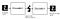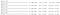source: https://blog.keras.io/building-autoencoders-in-keras.html

# Autoencoders in Computer Vision

## Buinding an Autoencodersource: https://blog.keras.io/building-autoencoders-in-keras.html
`from keras.datasets import mnistimport numpy as np(x_train, _), (x_test, _) = mnist.load_data()x_train = x_train.astype('float32') / 255.x_test = x_test.astype('float32') / 255.x_train = x_train.reshape((len(x_train), np.prod(x_train.shape[1:])))x_test = x_test.reshape((len(x_test), np.prod(x_test.shape[1:])))print(x_train.shape) #let's have a look at the shapeprint(x_test.shape)`
`import matplotlib.pyplot as pltplt.imshow(x_test.reshape(28, 28))plt.gray()`
`import kerasfrom keras import layersencoding_dim = 24  # 24 floats, it means that we have a compression factor of 784 (image input shape)/ 24 = 32.7input_img = keras.Input(shape=(784,))encoded = layers.Dense(encoding_dim, activation='relu')(input_img)decoded = layers.Dense(784, activation='sigmoid')(encoded)autoencoder = keras.Model(input_img, decoded)encoder = keras.Model(input_img, encoded)encoded_input = keras.Input(shape=(encoding_dim,))decoder_layer = autoencoder.layers[-1]decoder = keras.Model(encoded_input, decoder_layer(encoded_input))autoencoder.compile(optimizer='adam', loss='binary_crossentropy') #per-pixel binary crossentropy loss`
`autoencoder.fit(x_train, x_train,                epochs=50,                batch_size=256,                shuffle=True,                validation_data=(x_test, x_test))encoded_imgs = encoder.predict(x_test)decoded_imgs = decoder.predict(encoded_imgs)`…….
`plt.figure(figsize=(9, 4))plt.subplot(121)plt.imshow(x_test.reshape(28, 28))plt.gray()plt.subplot(122)plt.imshow(decoded_imgs.reshape(28, 28))plt.gray()`

## Conclusions

Cloud Specialist at @Microsoft | MSc in Data Science | Machine Learning, Statistics and Running enthusiast

## More from Valentina Alto

Cloud Specialist at @Microsoft | MSc in Data Science | Machine Learning, Statistics and Running enthusiast